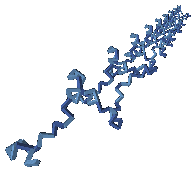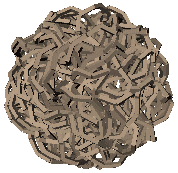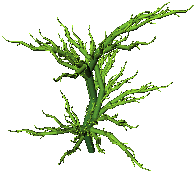## Fractal Robots

Back in the late 1990s, I needed a way to generate test cases for a new dynamics algorithm I was developing: the divide-and-conquer algorithm.  My solution to this problem was fractal robots, which I defined to be any rigid-body system in which at least one of the following was fractal: the connectivity, the geometry, or the configuration.  Some of these mechanisms turned out to be quite pretty, as you can see from the pictures below.  (Click on any image to see the full-size version.)

### TetraThis robot is an unbranched chain consisting of two types of link connected together by revolute joints.  All links have the same length, but one type has a skew angle (the angle between adjacent joint axes) of +α, whereas the other has a skew angle of −α (i.e., each type is the mirror image of the other).  The fractal appears in the sequence in which these two types of link occur along the chain.  Both the sequence and the value of α are based on the geometry of a tetrahedron.  The pictures above show this robot in two fractal configurations, the two types of link being drawn in slightly different shades of blue.  Each configuration is defined by two joint angles, θ1 and θ2, which repeat along the length of the chain in a fractal sequence closely related to the sequence of links.  The only difference between these pictures is the values of θ1 and θ2.  This robot is pretty, but it was never used for dynamics tests.

### BallThis robot is a simple chain of identical links connected by revolute joints.  Thus, there is nothing about the robot itself that is fractal.  However, the configuration is fractal, and is defined as follows: let θ1 and θ2 be the two angles that define the configuration, then the joint angles are set according to the sequence θ1, θ2, something, θ1, θ2, something, θ1, θ2, something, and so on, where the 'something's follow the exact same pattern (i.e., θ1, θ2, something, θ1, θ2, something, etc., recursively).  The two pictures above differ only in the values of θ1 and θ2.  The ball-shaped configuration gives the robot its name, and is the configuration used as a test case in DCA part 2.

### DillThis is a branched tree defined as follows: dill(n,α) is obtained by extending the first link of a dill(n−1,α) to accommodate one more joint, and attaching another dill(n−1,α) to that link via a revolute joint.  α is the skew angle between successive joint axes along a link.  This mechanism has some interesting properties relevant to the the divide-and-conquer algorithm, and was used as a test case in DCA part 2.